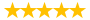# post university fin 201 unit 5 case problem 5

Question # 00004350 Posted By: spqr Updated on: 12/02/2013 01:03 AM Due on: 12/28/2013
Subject Finance Topic Finance Tutorials:
Question

Case Problem 5

Touring Enterprises, Inc., has a capital structure consisting of \$18 million in long-term debt and \$7 million in common equity. There is no preferred stock outstanding.

The interest rate paid on the long-term debt is 10%. The firm is in the 35% tax bracket.

On the common equity (stock), the Company pays an annual dividend of \$1.20 and expects to increase the dividend by 5% per year. The market price of the stock is \$50.

Based on this information, answer the following questions:

• Calculate Touring Enterprises' weighted average cost of capital (WACC).
• Work as follows: first, compute the after-tax cost of debt, then compute the cost of equity. Cite both formulas, and show all your work.
• Determine the weightings of debt and equity in the capital structure.
• 2. If Touring Enterprises were to increase the percentage of debt in its capital structure, what would happen to the WACC ? No calculation is necessary- simply provide a short, non-numeric response.
• 3. Identify and explain the benefits and risks of debt financing. A two-paragraph answer will suffice.

Hints

• Think of the WACC as follows: (weighting of debt * after-tax cost of debt) + (weighting of equity * cost of equity).
• Also, compute the costs of debt and equity in percentage terms from the very beginning. If you use decimals, you will have to deal with multiplying many decimals which can lead to confusion.
Tutorials for this Question
1. ## Solution: post university fin 201 unit 5 case problem 5

Tutorial # 00004146 Posted By: spqr Posted on: 12/02/2013 01:04 AM
Puchased By: 4
Tutorial Preview
non-numeric response.3. Identify and explain ...
Attachments
Case_Problem_5.xlsx (9.59 KB)
Recent Feedback
Rated By Feedback Comments Rated On
tr...irlHumble and supportive team 09/02/2014
jj...982Great in-depth tutorials 05/12/2014

Great! We have found the solution of this question!